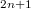﻿

### The contact Whitney sphere

#### Abstract

In this paper, we introduce the contact Whitney sphere as an imbedding of the$n$--dimensional unit sphere as an integral submanifold of the standard contact structure on$\R^{2n+1}$. We obtain a general inequality for integral submanifolds in$\R^{2n+1}$, involving both the scalar curvature and the mean curvature, and we use the equality case in order to characterize the contact Whitney sphere. We also study a similar problem foranti-invariant submanifolds of$\R^{2n+1}$, tangent to the structure vector field.

DOI Code: 10.1285/i15900932v20n2p125

Keywords: Integral submanifolds; Whitney spheres; Scalar curvature; Mean curvature

Classification: 53C15; 53C40; 53D10

Full Text: PDF